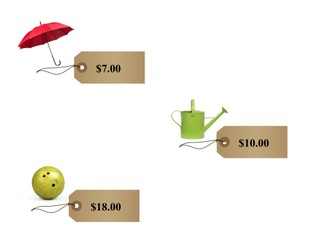Ordering numbers to 20

# Ordering numbers to 20

Ordering numbers to 20

No account needed.8,000 schools use Gynzy92,000 teachers use Gynzy1,600,000 students use Gynzy

## General

Students learn to set the numbers from 1 to 20 in order and know when a number is more or less.

-1.NBT.B.3

## Introduction

Have students form pairs and hand out number cards from 1 to 10 to each pair. They have to set the cards in order from least to most. To check that students can compare numbers to 20, practice as a class with a few examples on the interactive whiteboard. Ask students to explain their reasoning to determine if a number is more or less than another number. Check that students are making use of the number line.

## Instruction

Show the number line on the interactive whiteboard and explain that the numbers increase in value and that they have a specific order. Explain that you can set groups of objects in order from least to most. Do this by using the number sequence on the interactive whiteboard. Explain that you first look for the smallest number of the sequence. The next step is to find the second-smallest number. You keep going until all the numbers have been placed in order. Practice setting a few numbers from least to most as a class. Tell students that they can use the number line to help them with determining where a number belongs. Students then as a class practice with the number cards to 20 shown on the interactive whiteboard.

Check that students understand ordering numbers to 20 by asking the following questions:
- How do you set numbers in the right order?
- How do you know which number is the least?
- How do you know which number is the most?
- What can you use to help decide which order the numbers belong in?

## Quiz

Students first practice setting three numbers from least to most. Then they practice setting four numbers in the correct order.

## Conclusion

Discuss with the class that it is important to be able to set numbers in the correct order so you know what is more and less expensive. Check that students understand that they can use the number line as support and that they know how to set numbers in order from least to most. Check this by asking a few students to come to the front of the class and giving them number cards (to max 20). Have the class determine if they are in order from least to most or if they need to be moved. Have students explain their thinking.

## Teaching Tips

Students who have difficulty setting numbers in order can be helped by using the number line. By writing a number line to 20 and pointing (or marking) the numbers they have, they can see what the correct order should be.

## Instruction materials

Number cards from 1 to 20.

### The online teaching platform for interactive whiteboards and displays in schools

• Save time building lessons

• Manage the classroom more efficiently

• Increase student engagement